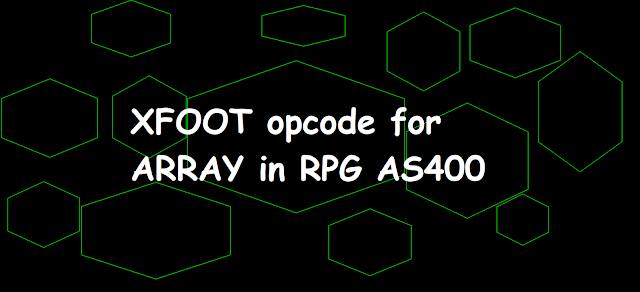XFOOT opcode for ARRAY in RPG AS400 - AS400 and SQL Tricks

# XFOOT opcode for ARRAY in RPG AS400

XFOOT opcode for ARRAY in RPG AS400XFOOT opcode for ARRAY in RPG AS400

## XFOOT in RPG Fixed Format

XFOOT opcode is used to sum all the numeric array elements.

### Coding XFOOT opcode to Sum array elements in Fixed Format RPGLE AS400

```     DArray            S              2P 0 DIM(5)
DSUM              S              4P 0
C                   EVAL      ARRAY(1) = 10
C                   EVAL      ARRAY(2) = 20
C                   EVAL      ARRAY(3) = 50
C                   EVAL      ARRAY(4) = 40
C                   EVAL      ARRAY(5) = 30
C                   XFOOT     ARRAY         SUM
C     SUM           DSPLY
C                   SETON                                        LR ```

### Output

`DSPLY  150`

## %XFOOT in RPG /Free and Fully Format

%XFOOT bif is used to sum all the array elements or specified multiple array elements.

`%XFOOT(array-expression)`

Please note that if there are two arrays having 5 and 10 elements respectively then %XFOOT(ARRAY1+ARRAY2) will result in summing up the first 5 elements of Array1 and Array2.

### Coding XFOOT opcode to Sum array elements in /Free and Fully Free RPGLE AS400

RPG Code in /Free format for XFOOT (Sum Array elements) in RPGLE AS400
```     DArray            S              2P 0 DIM(5)
DSUM              S              4P 0
/Free
ARRAY(1) = 10;
ARRAY(2) = 20;
ARRAY(3) = 50;
ARRAY(4) = 40;
ARRAY(5) = 30;
SUM = %XFOOT(ARRAY);

DSPLY SUM;
*INLR = *ON;
/End-Free
```
RPG Code in Fully Free format for XFOOT (Sum Array elements) in RPGLE AS400
```**FREE
ctl-opt debug(*yes);
ctl-opt Option(*NoDebugio);

dcl-s Array  packed(2:0) DIM(5);
dcl-s sum  packed(5:0);
ARRAY(1) = 10;
ARRAY(2) = 20;
ARRAY(3) = 50;
ARRAY(4) = 40;
ARRAY(5) = 30;
SUM = %XFOOT(ARRAY);

DSPLY SUM;
*INLR = *ON;
```